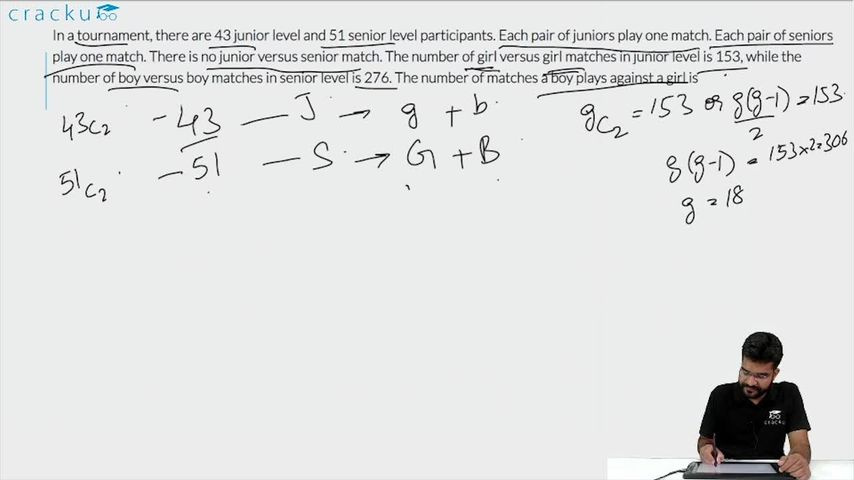Question 76

# In a tournament, there are 43 junior level and 51 senior level participants. Each pair of juniors play one match. Each pair of seniors play one match. There is no junior versus senior match. The number of girl versus girl matches in junior level is 153, while the number of boy versus boy matches in senior level is 276. The number of matches a boy plays against a girl is

Solution

In a tournament, there are 43 junior level and 51 senior level participants.

Let 'n' be the number of girls on junior level. It is given that the number of girl versus girl matches in junior level is 153.

$$\Rightarrow$$ nC2 = 153

$$\Rightarrow$$ n(n-1)/2 = 153

$$\Rightarrow$$ n(n-1) = 306

=> n$$^{2}$$-n-306 = 0

=> (n+17)(n-18)=0

=> n=18  (rejecting n=-17)

Therefore, number of boys on junior level = 43 - 18 = 25.

Let 'm' be the number of boys on senior level. It is given that the number of boy versus boy matches in senior level is 276.

$$\Rightarrow$$ mC2 = 276

$$\Rightarrow$$ m = 24

Therefore, number of girls on senior level = 51 - 24 = 27.

Hence, the number of matches a boy plays against a girl  = 18*25+24*27 = 1098

### View Video Solution